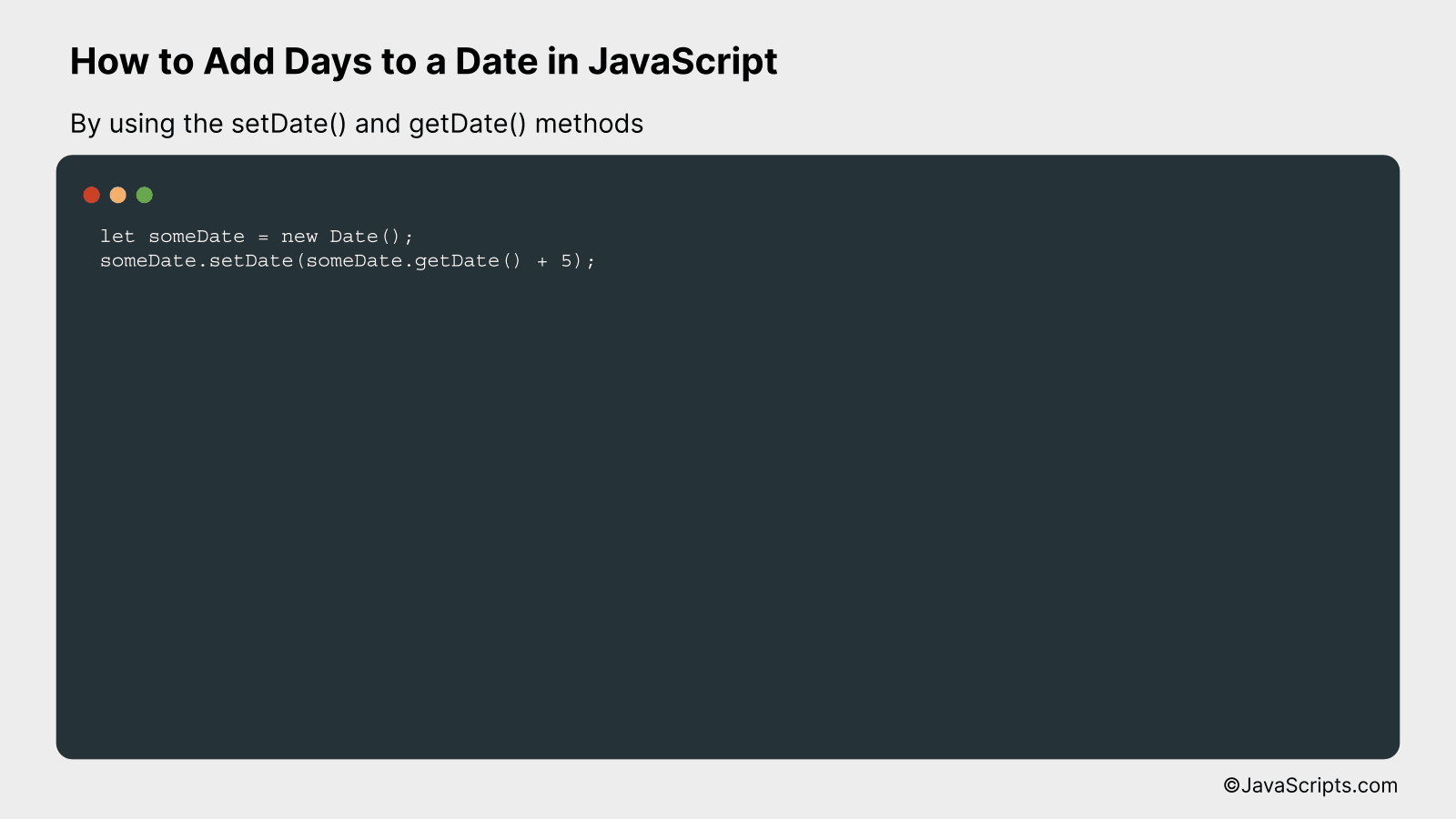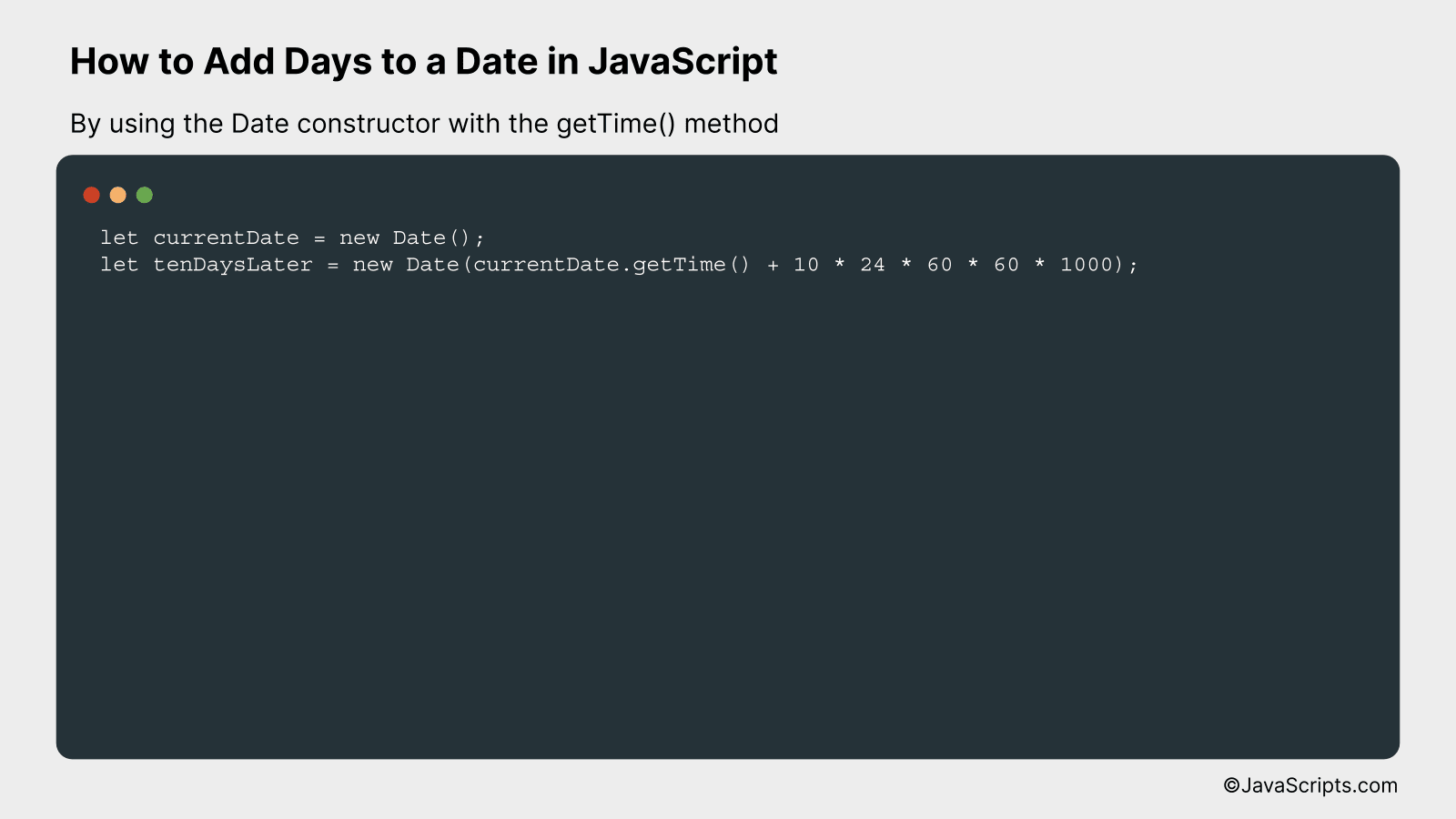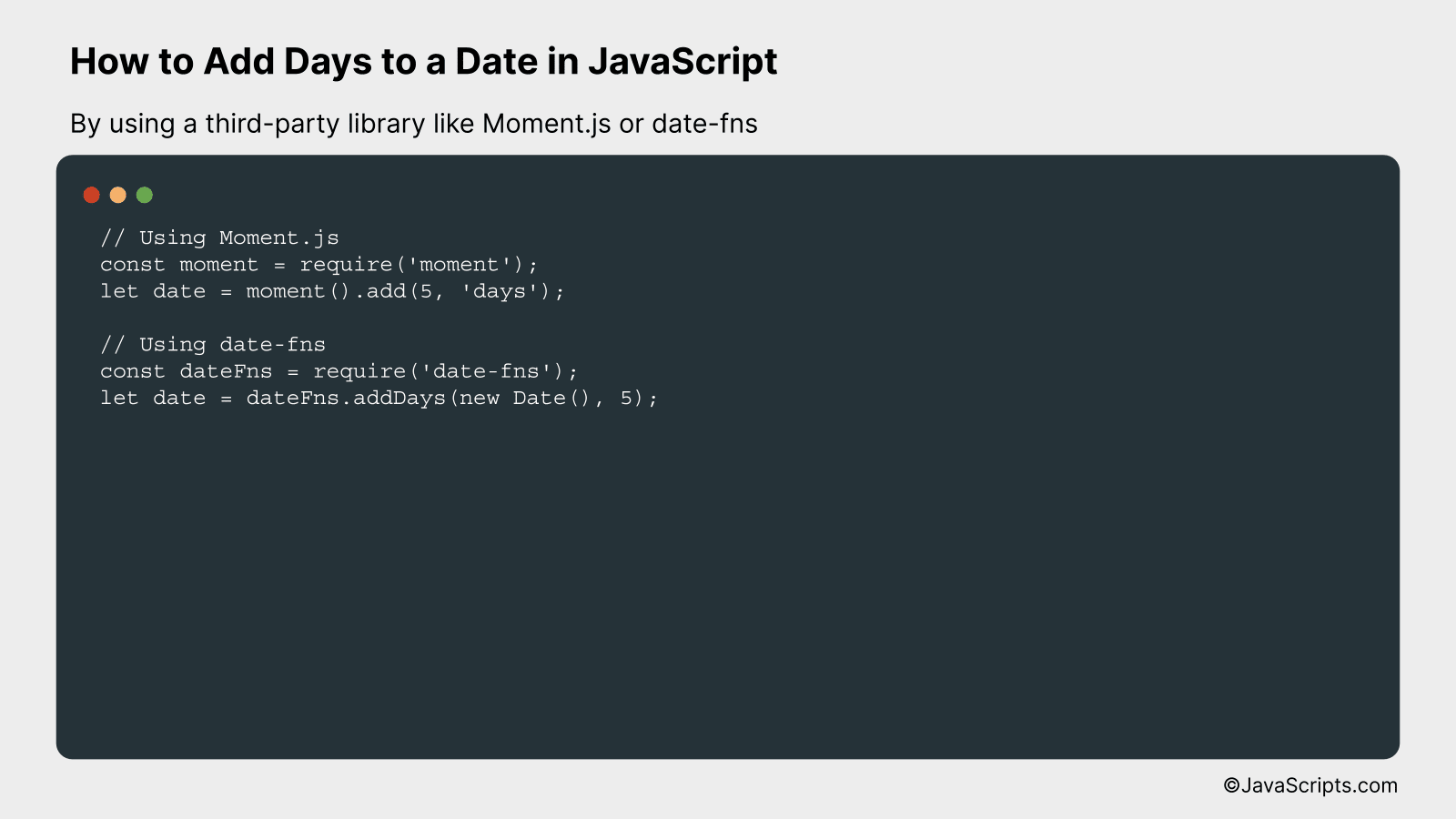# How to Add Days to a Date in JavaScript

Manipulating dates and times is often a necessity in programming, especially when you’re dealing with data scheduling or tracking tasks. But how do we smoothly add or subtract days from a date in JavaScript? It’s simpler than you might think.

We’ll guide you through this process step by step. You’ll see how JavaScript’s built-in Date object, along with some basic math, allows us to easily perform these operations. By the end, you’ll be handling date manipulations like a pro.

In this post, we will be looking at the following 3 ways to add days to a date in JavaScript:

• By using the setDate() and getDate() methods
• By using the Date constructor with the getTime() method
• By using a third-party library like Moment.js or date-fns

Let’s explore each…

## #1 – By using the setDate() and getDate() methodsThe JavaScript setDate() and getDate() methods can be used to add days to a date. This is accomplished by first getting the day from a date using getDate(), then adding the desired number of days, and setting the new date using setDate().

``````
let someDate = new Date();
someDate.setDate(someDate.getDate() + 5);
``````

### How it works

The setDate() method sets the day of the Date object relative to the beginning of the currently set month, while the getDate() method returns the day of the month from the Date object. By adding a number to the result of getDate(), we essentially “move” the date forward by that many days.

• First, a Date object is created with the current date and time using the ‘new Date()’ command.
• The getDate() method is then called on the ‘someDate’ object, which returns the current day of the month (from 1-31).
• We add the number of days we want to this returned value. In our example, we add 5 days.
• Finally, the setDate() method is called on the ‘someDate’ object again, setting the day of the month to the newly calculated value. This effectively “moves” the date forward by the desired number of days.

## #2 – By using the Date constructor with the getTime() methodThis process will involve creating a new JavaScript Date object and then using the getTime() method to retrieve the current timestamp. We will then add the desired number of days, converted to milliseconds, and construct a new Date object with this updated timestamp. For a clear understanding, let’s consider an example where we add 10 days to the current date.

``````
let currentDate = new Date();
let tenDaysLater = new Date(currentDate.getTime() + 10 * 24 * 60 * 60 * 1000);
``````

### How it works

The above code creates a new Date instance representing the current date and time. It then manipulates this timestamp to compute a new date that is 10 days later. The new timestamp is used to create a second Date instance representing the future date.

• Step 1: The Date constructor with no parameters, `new Date()`, creates a new Date object with the current date and time.
• Step 2: The `getTime()` method is called on this Date object. This method returns the number of milliseconds since the Unix Epoch (1 January 1970 00:00:00 UTC), representing the current timestamp.
• Step 3: To this timestamp, we add the number of milliseconds in 10 days. This is calculated as `10 * 24 * 60 * 60 * 1000`, which represents 10 days’ worth of milliseconds.
• Step 4: We use the updated timestamp to construct a new Date object, `new Date(currentDate.getTime() + 10 * 24 * 60 * 60 * 1000)`. This represents the date and time 10 days in the future.

## #3 – By using a third-party library like Moment.js or date-fnsThis guide will demonstrate how to add days to a date in JavaScript using Moment.js and date-fns libraries. You will be able to understand this better with an example where we add 5 days to the current date and then display the future date.

``````
// Using Moment.js
const moment = require('moment');

// Using date-fns
const dateFns = require('date-fns');
let date = dateFns.addDays(new Date(), 5);
```
```

### How it works:

The libraries Moment.js and date-fns provide a simple way to manage dates in JavaScript. In our code, we are adding 5 days to the current date using the add function in Moment.js and addDays in date-fns.

• The ‘moment()’ function in Moment.js and ‘new Date()’ in date-fns gets the current date and time.
• Then, we use the ‘add’ function in Moment.js and ‘addDays’ in date-fns to add a specific number of days to the current date.
• The ‘add’ function in Moment.js requires two parameters, the number of units to add and the type of unit (‘days’ in this case).
• The ‘addDays’ function in date-fns takes two parameters, the Date object to which days should be added, and the number of days to add.
• Finally, these functions return a new date object representing a future date.

Related:

In conclusion, adding days to a date in JavaScript is a simple and useful operation. As we’ve explored, the Date object and its methods make this task straightforward and efficient.

Remember, practice is key to mastering this skill. So, keep experimenting with different dates and number of days to add. Happy coding!